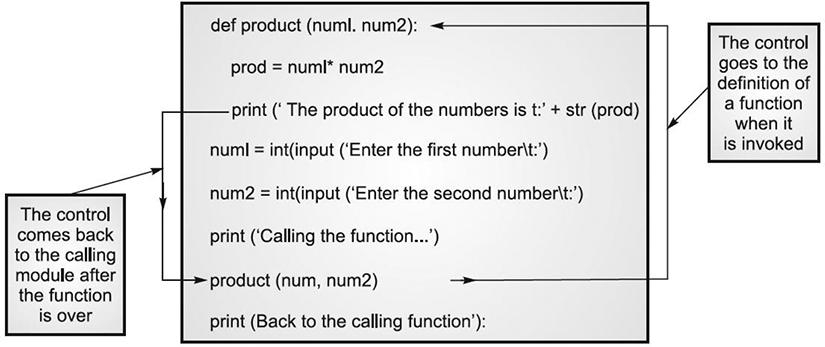# Python Function: How to Write Error Logic

Here's an example python user-defined function. Here, you'll know how to write error conditions. The best examples is if and else. I have shared the logic here for your reference.

Python
is one of the top programming languages. Python does support Functions. While writing functions you also need to consider some errors. Those are called exceptions. I am explaining how to give error logic in a function of Python.

Exception-handling means to have logic for error scenarios. Writing exception I will show in a simple Function.

In this post I will give you syntax for writing function and exception.

Here is my simple Function that multiplies two numbers.

Logic to write Function in Python to Multiply Two Numbers

def product (num1,num2):
prod=num1*num2
print( ' The product of the numbers is \t:
'+str(prod)

Parameters used the Python Function
• The 'def' means you are defining function with a name of 'product'.
• In the parameters of 'product', you can see TWO variables. Those are to take input from the user for multiplication.
• prod=num1*num2. That means product of two numbers assigning to 'prod' variable.
• Python is dynamic language and you no need to define variables explicitly.
• Print command displays your result to the terminal or console.
• The '\t' tells 'space' between comment and the result.
How Python Function worksHow to write error logic in Python Function

In the below code when you supply other than numeric, it sends an error message.

def product(num1,num2):
prod=num1*num2
If num1 or num2 in [ 0,1,2,3,4,5,6,7,8,9]
print( prints value)
elif
print( error)

Now, you are good to write functions and exceptions.

References